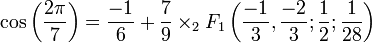# The Most Beautiful Equation?

Both of your choices, Entity, are my favourites!!!! I just love those equations and the first one, i understood only a few days ago!

So for me, they are the best equations!DaveC426913
Gold Member
Does the Mandelbrot Set count as an equation? It's really more of an algorithm I suppose.

2+2=5

2+2=5

How Orwellian of you.

2+2=5

:rofl:

Chi Meson
Homework Helper
2+2=5

That is only true for large values of 2.

pi=3.0

For me the Fourier transform equation is the most beautiful
$$F(\omega)=\int_{-\infty}^{\infty} f(t) e^{i \omega t} dt$$

That is only true for large values of 2.

As long as the error bars are sufficiently broad it could work as well.

hotvette
Homework Helper
I like:

x2+y2=1
y = xx

lisab
Staff Emeritus
Gold Member
pi=3.0

:tongue2:

178212+184112=192212Gold Member
pi=3.0

Upisoft hits R for 9000 damage (.14159 Overkill)
R dies

Upisoft hits R for 9000 damage (.14159 Overkill)
R dies

not over 9K, notice

Upisoft hits R for 9000 damage (.14159 Overkill)
R dies

They will fix it in the next version...:rofl:

xeniosm
the very simple (and suppressed!) standard model lagrangian

#### Attachments

•standardmodel.JPG
76.5 KB · Views: 415
$$f(x)=\frac{a_0}{2} + \sum_{n=1}^\infty \, [a_n \cos(nx) + b_n \sin(nx)]$$

I'm surprised no one has put this one up yet!my identity

a single equation? i dunno too many to think of

Last edited:
The most beautiful "equation" is

$$\langle a,b~\vert~o(a)=2, o(b)=3, o(ab)=29, o((ab)^4(abb)^2)=50, o(a((ab)^4(abb)^2)^{25})=5, o(ab^{abababababb})=34\rangle$$

Extra points for anybody who says what I've written down hereContainment
Ohm's law gets my vote.

Pengwuino
Gold Member
$$G_{\mu \nu} = 8T_{\mu \nu}$$

Where I have set $$c = G = \pi = 1$$

FtlIsAwesome
Gold Member
I'd say: 1 = 0. From this one, you can derive everything:rofl:

:tongue2: :tongue2: :tongue2:

I find derivatives beautiful even though I also hate them.

Pythagorean
Gold Member
Math:

Euler's Equation
$$e^{\pm i \theta} = \cos(\theta) + i \sin(\theta)$$

because it demonstrates the meaning of i as a transform/operator, rather than sqrt(-1)

Physics:

Continuity Equation (for conserved $$\phi$$):
$$\frac{\delta \phi}{\delta t} + \nabla \cdot f = 0$$

because it relates the physical meaning of the partial derivative to that of the total derivative.

Biology:

$$\Delta G = -n F \Delta E$$

Energy for work is the number of moles of stored energy from electric charge.